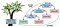# 10 Classification Methods From Scikit Learn We Should Know

`1. Number of times pregnant2. Plasma glucose concentration a 2 hours in an oral glucose tolerance test3. Diastolic blood pressure (mm Hg)4. Triceps skin fold thickness (mm)5. 2-Hour serum insulin (mu U/ml)6. Body mass index (weight in kg/(height in m)^2)7. Diabetes pedigree function8. Age (years)9. Class variable (0 or 1)`
`df = spark.table ('pima_indians_diabetes')print(f"""There are {df.count()} records in the dataset.""")df.show(5)`
`import pandas as pddataset = df.toPandas()`

# Data Analysis

## Mean & Standard Deviation

`dataset.describe().transpose()`

## Scatter Matrix

`sampled_data = df.drop ("Class").sample(False, 0.8).toPandas()axs = pd.plotting.scatter_matrix(sampled_data, figsize=(10, 10))num_cols = len(sampled_data.columns)for cur_col in range(num_cols):    ax = axs[cur_col, 0]    ax.yaxis.label.set_rotation(0)    ax.yaxis.label.set_ha('right')    ax.set_yticks(())    h = axs[num_cols-1, cur_col]    h.xaxis.label.set_rotation(90)    h.set_xticks(())`

# Data Preprocessing

## Train & Test Datasets

`X = dataset.iloc[:,:-1].valuesy = dataset.iloc[:, -1].values`
`from sklearn.model_selection import train_test_splitX_train, X_test, y_train, y_test = \        train_test_split( \                         X, y, \                         test_size = 0.25, \                         random_state = 0)`

## Standard Scaling

`from sklearn.preprocessing import StandardScalersc = StandardScaler()X_train = sc.fit_transform(X_train)X_test = sc.transform(X_test)`

# 1. Logistic Regression

## Implementation

`from sklearn.linear_model import LogisticRegressionlr = LogisticRegression(random_state = 0)lr.fit(X_train, y_train)`
`y_pred_lr = lr.predict(X_test)`
`from sklearn.metrics import confusion_matrix, accuracy_scorecm_lr = confusion_matrix(y_test, y_pred_lr)print(cm_lr)acc_lr = accuracy_score(y_test, y_pred_lr)print (acc_lr)`

# 2. K-Nearest Neighbours (K-NN)

## Implementation

`from sklearn.neighbors import KNeighborsClassifierknn = KNeighborsClassifier(n_neighbors = 5, \                           metric = 'minkowski', p = 2)knn.fit(X_train, y_train)`
`y_pred_knn = knn.predict(X_test)`
`from sklearn.metrics import confusion_matrix, accuracy_scorecm_knn = confusion_matrix(y_test, y_pred_knn)print (cm_knn)acc_knn = accuracy_score(y_test, y_pred_knn)print (acc_knn)`

# 3. SVC (Support Vector Classifier) with Linear Kernel

## Implementation

`from sklearn.svm import SVCsvc = SVC(kernel = 'linear', random_state = 0)svc.fit(X_train, y_train)`
`y_pred_svc = svc.predict(X_test)`
`from sklearn.metrics import confusion_matrix, accuracy_scorecm_svc = confusion_matrix(y_test, y_pred_svc)print (cm_svc)acc_svc = accuracy_score(y_test, y_pred_svc)print (acc_svc)`

# 4. Kernel SVM (Support Vector Machine)

## Implementation

`from sklearn.svm import SVCsvc_rbf = SVC(kernel = 'rbf', random_state = 0)svc_rbf.fit(X_train, y_train)`
`y_pred_svc_rbf = svc_rbf.predict(X_test)`
`from sklearn.metrics import confusion_matrix, accuracy_scorecm = confusion_matrix(y_test, y_pred_svc_rbf)print (cm)acc_svc_rbf = accuracy_score(y_test, y_pred_svc_rbf)print (acc_svc_rbf)`

# 5. Naïve Bayes

## Implementation

`from sklearn.naive_bayes import GaussianNBnb = GaussianNB()nb.fit(X_train, y_train)`
`y_pred = nb.predict(X_test)`
`from sklearn.metrics import confusion_matrix, accuracy_scorecm = confusion_matrix(y_test, y_pred)print(cm)acc_nb = accuracy_score(y_test, y_pred)print(acc_nb)`

# 6. Decision TreeImage source: Learning Spark, 2nd Edition, figure 10–9. Decision tree example.

## Implementation

`from sklearn.tree import DecisionTreeClassifierdt = DecisionTreeClassifier(criterion = 'entropy', random_state = 0)dt.fit(X_train, y_train)`
`y_pred = dt.predict(X_test)`
`from sklearn.metrics import confusion_matrix, accuracy_scorecm = confusion_matrix(y_test, y_pred)print (cm)acc_dt = accuracy_score(y_test, y_pred)print (acc_dt)`

# 7. Random Forest

## Implementation

`from sklearn.ensemble import RandomForestClassifierrf = RandomForestClassifier(max_depth=2, random_state=0)rf.fit(X_train, y_train)`
`y_pred_rf = rf.predict(X_test)`
`from sklearn.metrics import confusion_matrix, accuracy_scorecm = confusion_matrix(y_test, y_pred_rf)print (cm)acc_rf = accuracy_score(y_test, y_pred_rf)print (acc_rf)`

## Implementation

`from sklearn.ensemble import AdaBoostClassifierabc = AdaBoostClassifier()abc.fit(X_train, y_train)`
`y_pred_abc = abc.predict(X_test)`
`from sklearn.metrics import confusion_matrix, accuracy_scorecm = confusion_matrix(y_test, y_pred_abc)print (cm)acc_abc = accuracy_score(y_test, y_pred_abc)print (acc_abc)`

## Implementation

`from sklearn.discriminant_analysis \     import QuadraticDiscriminantAnalysisqda = QuadraticDiscriminantAnalysis()qda.fit(X_train, y_train)`
`y_pred_qda = qda.predict(X_test)`
`from sklearn.metrics import confusion_matrix, accuracy_scorecm = confusion_matrix(y_test, y_pred_qda)print (cm)acc_qda = accuracy_score(y_test, y_pred_qda)print (acc_qda)`

# 10. MLP Classifier

## Implementation

`from sklearn.neural_network import MLPClassifiermlp = MLPClassifier(alpha=1, max_iter=1000)mlp.fit(X_train, y_train)`
`y_pred_mlp = mlp.predict(X_test)`
`from sklearn.metrics import confusion_matrix, accuracy_scorecm = confusion_matrix(y_test, y_pred_mlp)print (cm)acc_mlp = accuracy_score(y_test, y_pred_mlp)print (acc_mlp)`

Written by